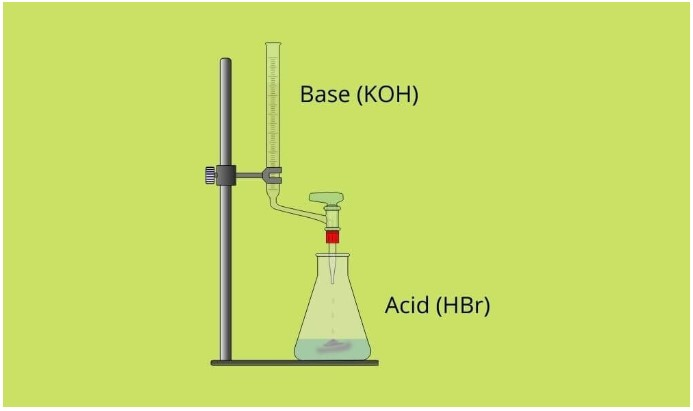# Neutralization Reaction Calculator

To find the equivalent weight of acid or base in a chemical solution, use the acid-base aka neutralization calculator.

Formula Normality = Weight Volume * Equivalent Weight

Give Us Feedback

The neutralization calculator finds the weight of acid or base that will react with hydrogen or hydroxyl ions i.e equivalent weight.

This calculator uses the normality of solution, volume, and weight of acid or base in the solution to compute equivalent weight.

## What is neutralization?

Neutralization is a chemical process of making salt and water using acid and base as reactants.

This reaction is supported by the fact that 1g of the base is completely neutralized by 1g of the acid.

For example:

HBr (Acid) +  KOH (Base)  =  KBr (salt)   +      H20  (water)## Examples of neutralization

Some other examples of neutralization are

• HNO3      +      KOH    →     KNO3     +    H2
• NaOH     +      H2SO4  →     Na2SO4   +    H2O
• Mg(OH)2 +      2HCL   →    MgCl2     +    2H2

## Equivalent weight formula

The equation of equivalent weight:

Equivalent weight = weight / (volume × normality)

The weight and volume are of the acid or base under study.

## How is equivalent weight important?

Aside from neutralization, calculating equivalent weight can be useful in different fields of chemistry.

1. Volumetric analysis (To find heavier compounds to reduce errors.)
2. Gravimetric analysis
3. Polymer analysis (reactivity of polyol, isocyanate e.t.c)

## How to calculate equivalent weight?

Example

What will be the equivalent weight of base if its weight is 30 grams, volume is 50 and normality is 20.

Solution:

Step 1: Write the given values.

Weight of base = 30 g

Volume = 50

Normality = 20

Step 2: Place the values in the formula.

Equivalent weight = 30 / (50 ×  20)

= 0.03

### Math Tools